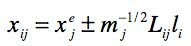## Wednesday, June 16, 2010

### Fold.it - Solve puzzles for science

I just came across this amazing game/puzzle/software - call it what you will - Foldit.

It's an interactive protein folder that evaluates the energy on the fly to let you know how well you are doing.

But, and here is the important bit, the energy function is the same used in the Rosetta packages - a state-of-the-art protein folder (at least that's how I read it - the details are a bit sketchy). This is not a toy, but an exciting experiment to test whether human intuition and pattern recognition can compete with Monte Carlo sampling.

The screencast below (see a bigger version here) shows 6 intro puzzles that teaches you some of the techniques for protein folding. You get the program here and make an account for yourself here (making an account using Foldit did not work for me).

When you start Foldit the first time it takes you into the intro puzzles immediately (i.e. bypassing the menu).

I don't know where to start about the many implications of this program for fundamental science, teaching, and public outreach. So I'll simply say wow!, and go on to level 2-3. Not a moment to lose, CASP9 is underway you know!

June 20th, 2010 update:

Not sure why I didn't think to check youtube immediately but there are many videos about foldit. Here are a couple:

## Monday, June 7, 2010

### Geometry and molecular motionFigure 4.18. The relative energy of H2 as a function of H–H distance and the energy of the lowest vibrational level (horizontal line).
From Molecular Modeling Basics CRC Press, 2010

When discussing structure and other molecular properties it is important to remember that the molecule is in constant internal motion due to vibrational motion. The bond length and angles that one so carefully computes and reports to so many decimal places actually changes considerably with time.

Consider the H2 molecule. Using B3LYP/6-31G(d) and the harmonic oscillator approximation, the frequency for the H–H stretch vibration is 4450 cm–1, which corresponds to 6.4 kcal/mol of kinetic energy. If we compute the energy as a function of the H–H bond length (Figure 4.18), we see that this kinetic energy is enough to compress the H–H bond length to roughly 0.64 Å and increase it to 0.88 Å: the classical turning points.

The classical turning points can be estimated using the harmonic approximation of the potential energy surface (PES)where νi is the vibrational frequency in units of cm–1, with a corresponding normal coordinate li (see section 1.3 of the book), and n is the vibrational quantum number. Thus, νi = 4450 cm–1 corresponds to a turning point at li =±0.087 amu½ × Å which can be converted to Cartesian coordinates (and hence a bond length) using the normal mode component LijIn the case of H2, this gives classical turning points at 0.621 Å and 0.865 Å, which are in good agreement with the previous estimate from Figure 4.18. The screencast below shows ho MacMolPlt can be used to estimate the classical turning points.

For lower frequencies the displacements at the classical turning points can be quite substantial, both because the PES is flatter and because higher vibrational levels are populated. For example, in the case of ethane the lowest frequency is 310 cm–1 [at the B3LYP/6-31G(d) level of theory] and corresponds mainly to rotation about the CC bond (Figure 4.20a). For the ground vibrational level the classical turning points occur at li =±0.330 amu½ × Å , which corresponds to a dihedral angle change of ±14° (Figure 4.20b, see screencast below). At room T 4% of the ethane molecules have three quanta of energy in this mode (n = 2), corresponding to a kinetic energy of 2.2 kcal/mol, enough to change the dihedral angle by ±31°.

Figure 4.20. (a) Normal mode associated with the lowest frequency computed for ethane using B3LYP/6-31G(d). (b) Structure obtained by displacing along the mode by 0.330.
Click on the picture for an interactive version.
From Molecular Modeling Basics CRC Press, 2010

Some of the lowest frequencies you’ll observe are for intermolecular interactions such as the water dimer hydrogen bond (Figure 4.22). Curious how much the geometry is displaced? Why not try it yourself? (See this post to get started with GAMESS).

Figure 4.22. (a) The PM3 normal mode corresponding to (a) torsional motion about the hydrogen bond and (b) hydrogen bond stretch in the water dimer. The corresponding frequencies are 128 and 415 cm–1.
Click on the picture for an interactive version.